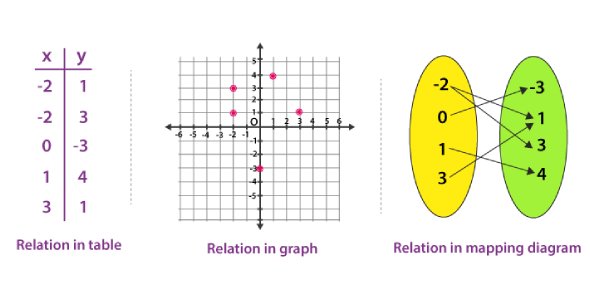# JEE Main Quiz: Sets, Relation And Functions Set 2

10 Questions | Attempts: 129
ShareSettings.

• 1.
A survey shows that 60% of Indians like football whereas 76% like cricket. If X% of the Indians like both mangoes and apples, then:
• A.

15 ≤ x ≤ 19

• B.

9 ≤ x ≤ 19

• C.

6 ≤ x ≤ 7

• D.

3 ≤ x ≤ 5

• 2.
Let A = {a, b, c, d}. The total number of unordered pairs of disjoint subsets of A is equal to:
• A.

An ellipse

• B.

A pair of straight

• C.

A hyperbola

• D.

A parabola

• 3.
Two points X and Y in a plane are related if OX = OY, where o is a fixed point. This relation is:
• A.

Partial Order Relation

• B.

Equivalence relation

• C.

Reflexive but not symmetric

• D.

Reflexive but not transitive

• 4.
• A.

A is Reflexive, symmetric and transitive.

• B.

A is Reflexive, transitive but not symmetric

• C.

A is Symmetric, Transitive but not reflexive

• D.

A is neither transitive nor reflexive but symmetric

• 5.
In a town of 10,000 families, it was found that 40% families buy newspaper A, 20% families buy newspaper B and 10% families buy newspaper C. 5% families buy A & B, 3% buy B & C and 4% buy c and A. If 2% of families buy all the newspaper, then how many families buy newspaper A only?
• A.

3300

• B.

4100

• C.

2600

• D.

3700

• 6.
• A.

Reflexive but not symmetric

• B.

Reflexive but not transitive

• C.

Symmetric and transitive

• D.

Equivalence relation

• 7.
• A.

A = B

• B.
• C.
• D.

None of these

• 8.
Let A & B are two finite sets having x and y no of elements respectively. Then the total no of mappings from A to B is:
• A.
• B.

Xy

• C.
• D.
• 9.
• A.

– f(x)

• B.

3f(x)

• C.
• D.

None of these

• 10.
Let X be a set containing 10 distinct elements, then the total number of distinct functions from X to X is:
• A.

100

• B.
• C.
• D.

## Related TopicsBack to top
×

Wait!
Here's an interesting quiz for you.